# 6.837 Intro to Computer Graphics Assignment 0: Iterated Function Systems

The goal of this assignment is to get familiar with C++ and with two simple libraries that we will use for linear algebra and images. The incidental goal is also to have fun with bizarre fractal objects. IFS are self-similar fractals: a subpart of the object is similar to the whole. The classic example of an IFS is Barnsley's fern, where each subpart of the fern is exactly the same as the whole fern. IFS are described by a set of affine transformations (rotations, translations, scale, skew, etc.) These transformations capture the self-similarity of the object. IFS can be defined in any dimension, but we will play with two-dimensional ones. Formally, an IFS is defined by n affine transformations. Each transformation fi must be contractive: The distance between points must be reduced. An attractor of the IFS is the object such that A = U fi (A). A is unchanged by the set of transformations: It is a fixed point.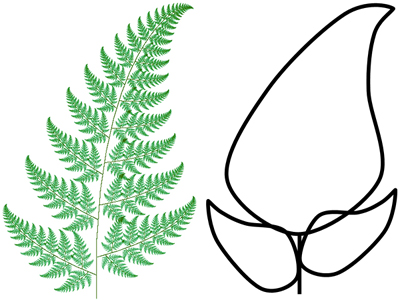We render an IFS by iterating the transform on random input points from the unit square. We approximate the fixed point by applying the transformation many times. The algorithm is as follows:

```   for "lots" of random points (x0, y0)
for k=0 to num_iters
pick a random transform fi
(xk+1, yk+1) = fi(xk, yk)
display a dot at (xk, yk)
```

To reduce the number of points necessary to make an image of reasonable quality, probabilities are assigned to each transformation, instead of choosing a transformation with uniform probability.

• Write a C++ class IFS that renders iterated function systems, including the interface (in a file ifs.h) and the implementation (ifs.C). Your code should run under Linux or Windows. The IFS class should include:
• a field to store n, the number of transformations
• an array of matrices representing the n transformations
• an array of the corresponding probabilities for choosing a transformation
• a constructor that creates an IFS
• an input method that reads the IFS description
• a render method that takes as input an image instance, a number of points and a number of iterations
• a destructor that frees the memory of the various arrays (using delete)
• Write the main program main.C that creates an Image instance, reads an IFS description from a file, renders the IFS to the image, and saves the image.
• Use the linear algebra library for the point and transformation representations.
• Perform proper memory management --- free memory when an object is destroyed.
• Extra credit: create a new IFS, figure out the probabilities, determine the bounding box, change the color scheme, anti-aliasing, depth-first vs. breadth-first, etc. Include a short paragraph in your README.txt file describing your extensions.

### Hints

• Random numbers can be obtained using the drand48() or rand(), and RAND_MAX. See stdlib.h.
• To debug your code, set the number of iterations to one. This will allow you to check that you got the transformations right.
• Be careful, arrays are indexed from 0 to n-1 in C++. Reading beyond the bounds of the array will probably result in a segmentation fault.
• Use assert() to check function pre-conditions, array indices, etc. See assert.h.

### Image Library

The Image class is used to initialize and edit the rgb values of images. Be careful --- do not try to edit values outside the bounds of the image. The class also includes functions for loading and saving simple .tga image files. .tga files can be viewed with xv or opened in Photoshop and other Windows image viewers/editors.

### Linear Algebra Library

Linear algebra support for floating point vectors with 2, 3, and 4 elements (Vec2f, Vec3f, and Vec4f) and 4x4 floating point matrices (Matrix). For this assignment, the void Matrix::Transform(Vec2f &v) function will be handy.

### Sample code for parsing command-line arguments and input files

Your program should take a number of command line arguments to specify the input file, number of points, number of iterations, output image size and output file. Make sure the examples below work, as this is how we will test your program. Sample code to parse input files and command line arguments is provided:

### Data files

The input data for an IFS is a file which contains n, the number of transforms, followed by the probability of choosing each transform and a 3x3 floating point matrix representation of the transform.

### Sample Results

```ifs -input sierpinski_triangle.txt -points 10000 -iters 0 -size 200 -output sierpinski_triangle_0.tga
ifs -input sierpinski_triangle.txt -points 10000 -iters 1 -size 200 -output sierpinski_triangle_1.tga
ifs -input sierpinski_triangle.txt -points 10000 -iters 2 -size 200 -output sierpinski_triangle_2.tga
ifs -input sierpinski_triangle.txt -points 10000 -iters 3 -size 200 -output sierpinski_triangle_3.tga
ifs -input sierpinski_triangle.txt -points 10000 -iters 4 -size 200 -output sierpinski_triangle_4.tga
ifs -input sierpinski_triangle.txt -points 10000 -iters 30 -size 200 -output sierpinski_triangle.tga
```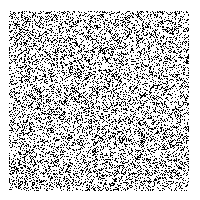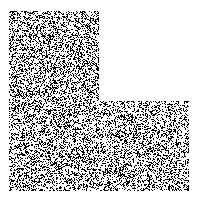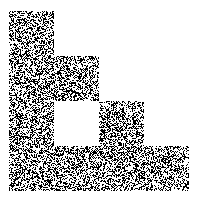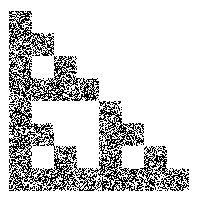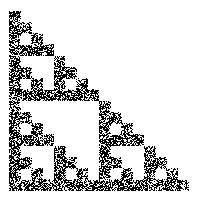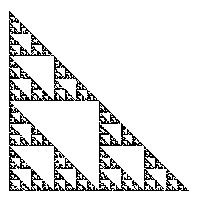```ifs -input fern.txt -points 50000 -iters 30 -size 400 -output fern.tga
```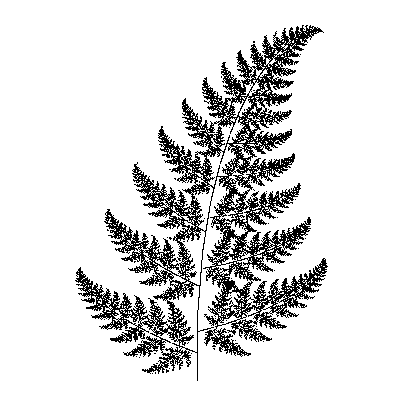See the main Assignments Page for submission information.